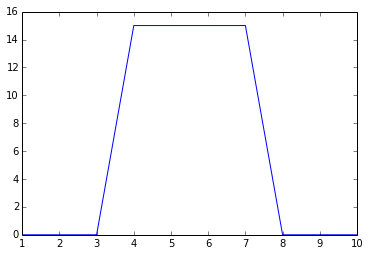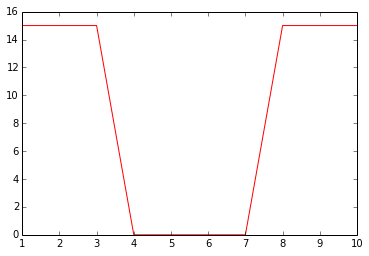## Example 14-1, Page 481¶

In :
VGS_off=-3.0            #Gate-source cutoff voltage(V)
IDSS=6                  #IDSS current(mA)
VGS1=-1                 #VGS voltage(V)
VGS2=-2
VGS3=0
VGS4=1
VGS5=2

ID1=IDSS*((1-(VGS1/VGS_off))**2)
ID2=IDSS*((1-(VGS2/VGS_off))**2)
ID3=IDSS*((1-(VGS3/VGS_off))**2)
ID4=IDSS*((1-(VGS4/VGS_off))**2)
ID5=IDSS*((1-(VGS5/VGS_off))**2)

print 'Drain current ID1 = ',round(ID1,2),'mA'
print 'Drain current ID2 = ',round(ID2,2),'mA'
print 'Drain current ID3 = ',ID3,'mA'
print 'Drain current ID4 = ',round(ID4,2),'mA'
print 'Drain current ID5 = ',round(ID5,2),'mA'

Drain current ID1 =  2.67 mA
Drain current ID2 =  0.67 mA
Drain current ID3 =  6.0 mA
Drain current ID4 =  10.67 mA
Drain current ID5 =  16.67 mA


## Example 14-2, Page 482¶

In :
VGS_off=-2.0            #Gate-source cutoff voltage(V)
IDSS=4                  #IDSS current(mA)
gm0=2000*10**-6         #transconductance(S)
VGS=0                   #VGS voltage(V)
VDD=15                  #Drain supply voltage(V)
RD=2.0                  #Drain resistance(KOhm)
Vin=20                  #input voltage(mV)

VDS=VDD-(IDSS*RD)       #Drain-source voltage(V)
rd=RD*RL/(RD+RL)        #ac drain resistance(KOhm)
Av=gm0*rd*1000          #voltage gain
Vout=Vin*Av             #output voltage (mV)

print 'Output voltage Vout = ',round(Vout,2),'mV'

Output voltage Vout =  66.67 mV


## Example 14-3, Page 490¶

In :
VGS_on=4.5              #Gate-source voltage(V)
ID_on=75                #ID current(mA)
RDS=6                   #Ohmic resistance(Ohm)
VDD=20                  #Drain supply voltage(V)
RD=1.0                  #Drain resistance(KOhm)

ID_sat=VDD/RD                  #drain saturation current(mA)
Vout1=VDD*(RDS/(RDS+(RD*1000)))#output voltage when VGS is high(V)
Vout2=VDD                      #output voltage when VGS is low(V)

print 'output voltage when VGS is high = ',round(Vout1,2),'V'
print 'output voltage when VGS is low = ',Vout2,'V'

output voltage when VGS is high =  0.12 V
output voltage when VGS is low =  20 V


## Example 14-4, Page 492¶

In :
ID=20                     #ID current as per previous example(mA)
Vled=2                    #Led drop(V)
RD=1.0                    #Drain resistance(KOhm)

ID=(VDD-Vled)/RD          #drain current considering LED drop

print 'drain current considering LED drop ID = ',ID,'mA'

drain current considering LED drop ID =  18.0 mA


## Example 14-5, Page 492¶

In :
Rrc=500.0          #Resistance of relay(V)
VDD=24             #drain supply voltage(V)

ID_sat=VDD/Rrc     #drain saturation current(A)

print 'Drain saturaion current ID(sat) = ',ID_sat*1000,'mA'

Drain saturaion current ID(sat) =  48.0 mA


## Example 14-6, Page 496¶

In :
VDD=20.0                    #drain supply voltage (V)
RD=10*10**3                 #drain resistance(Ohm)
RDS=50.0                    #Ohmic resistance(Ohm)

Vout1=VDD                   #Output voltage when i/p is low (V)
Vout2=VDD*RDS/(RDS+RD)      #Output voltage when i/p is high (V)

print 'Output voltage when input is low Vout1 = ',Vout1,'V'
print 'Output voltage when input is high Vout2 = ',round((Vout2*1000),2),'mV'

Output voltage when input is low Vout1 =  20.0 V
Output voltage when input is high Vout2 =  99.5 mV


## Example 14-7, Page 496¶

In :
VDD=10.0                     #drain supply voltage (V)
RD=2*10**3                   #drain resistance(Ohm)
RDS=500.0                    #Ohmic resistance(Ohm)

Vout1=VDD                   #Output voltage when i/p is low (V)
Vout2=VDD*RDS/(RDS+RD)      #Output voltage when i/p is high (V)

print 'Output voltage when input is low Vout1 = ',Vout1,'V'
print 'Output voltage when input is high Vout2 = ',Vout2,'V'

Output voltage when input is low Vout1 =  10.0 V
Output voltage when input is high Vout2 =  2.0 V


## Example 14-8, Page 498¶

In :
import matplotlib.pyplot as plt
%matplotlib inline

print 'Input signal switches from 0 to 15 V at pt. A & 15 to 0 V at pt. B'
x=[1,2,3,4,5,6,7,8,9,10]
y=[0,0,0,15,15,15,15,0,0,0]
print 'approximate plotting shown in figure'
plt.plot(x,y)
plt.show()
print 'output signal shown on second plot'
z=[15,15,15,0,0,0,0,15,15,15]
plt.plot(x,z,'r')
plt.show()

Populating the interactive namespace from numpy and matplotlib
Input signal switches from 0 to 15 V at pt. A & 15 to 0 V at pt. B
approximate plotting shown in figureoutput signal shown on second plot## Example 14-9, Page 502¶

In :
VDD=30.0                    #drain supply voltage (V)
RD=30                       #drain resistance(Ohm)
RDS=1.95                    #Ohmic resistance(Ohm)
ID_on=2                     #ID for MOSFET on (A)

ID_sat=VDD/RD                    #drain saturation current(A)
ID=VDD/(RDS+RD)                  #drain current(A)

print 'current through motor winding is ID = ',round(ID,2),'A'

current through motor winding is ID =  0.94 A


## Example 14-10, Page 503¶

In :
VDD=30.0                    #drain supply voltage (V)
RD=10                       #drain resistance(Ohm)
RDS=1.07                    #Ohmic resistance(Ohm)
ID_on=5                     #ID for MOSFET on (A)

ID_sat=VDD/RD                    #drain saturation current(A)
ID=VDD/(RDS+RD)                  #drain current(A)

print 'current through lamp is ID = ',round(ID,2),'A'

current through lamp is ID =  2.71 A


## Example 14-11, Page 503¶

In :
VDD=10.0                    #drain supply voltage (V)
RD=10                       #drain resistance(Ohm)
RDS=0.5                     #Ohmic resistance(Ohm)
ID_on=5                     #ID for MOSFET on (A)

ID=VDD/(RDS+RD)             #drain current(A)

print 'current through water valve is ID = ',round(ID,2),'A'

current through water valve is ID =  0.95 A


## Example 14-12, Page 504¶

In :
VDD=30.0                    #drain supply voltage (V)
RD=10                       #drain resistance(Ohm)
RDS=1.07                    #Ohmic resistance(Ohm)
R1=2*10**6                  #Resistance1 at gate (Ohm)
R2=1*10**6                  #Resistance2 at gate (Ohm)
C=10*10**-6                 #Capacitance at gate(F)

RTH=R1*R2/(R1+R2)           #Thevenin resistance(Ohm)
RC=RTH*C                    #RC Time constant(s)
ID=VDD/(RDS+RD)             #drain current through lamp(A)
P=(ID**2)*RD                #Lamp Power(W)

print 'RC Time constant = ',round(RC,2),'s'
print 'current through lamp is ID = ',round(ID,2),'A'
print 'Lamp power P = ',round(P,2),'W'

RC Time constant =  6.67 s
current through lamp is ID =  2.71 A
Lamp power P =  73.44 W


## Example 14-13, Page 506¶

In :
ID_on=0.6                   #ID for MOSFET on (A)
VGS_on=4.5                  #VGS for MOSFET on(V)
VGS_th=2.1                  #VGS threshold(V)
VGS1=3                      #Gate-source voltage1(V)
VGS2=4.5                    #Gate-source voltage2(V)

k=ID_on/(VGS_on-VGS_th)**2  #constant(A/V2)
ID1=1000*k*(VGS1-VGS_th)**2      #ID for VGS=3 (mA)
ID2=1000*k*(VGS2-VGS_th)**2      #ID for VGS=4.5 (mA)

print 'ID1 (for VGS = 3V) = ',round(ID1,2),'mA'
print 'ID2 (for VGS = 4.5V) = ',ID2,'mA'

ID1 (for VGS = 3V) =  84.37 mA
ID2 (for VGS = 4.5V) =  600.0 mA


## Example 14-14, Page 507¶

In :
ID_on=3                 #ID (On) (mA)
VDS_on=10               #VDS(On) (V)
VDD=25                  #Drain supply voltage (V)

RD=(VDD-VDS_on)/ID_on   #Drain resistance(KOhm)

print 'Drain resistance RD = ',RD,'KOhm'

Drain resistance RD =  5 KOhm


## Example 14-15, Page 508¶

In :
ID_on=600               #ID (On) (mA)
VGS_th=2.1              #VGS(threshold) (V)
VDD=12                  #Drain supply voltage (V)
k=104*10**-3            #constant(A/V2)
R1=1.0*10**6            #Resistance1 at gate (Ohm)
R2=350.0*10**3          #Resistance2 at gate (Ohm)
Vin=100.0*10**-3        #input voltage (V)
RD=68.0                 #Drain resistance(Ohm)

VGS=VDD*R2/(R1+R2)              #Gate-source voltage(V)
ID=1000*k*(VGS1-VGS_th)**2      #Drain current (mA)
gm=2*k*(VGS-VGS_th)             #transconductance (S)
rd=RD*RL/(RD+RL)                #ac drain resistance(Ohm)
Av=gm*rd                        #voltage gain
Vout=Vin*Av                     #Output voltage (V)

print 'Gate-source voltage VGS = ',round(VGS,2),'V'
print 'transconductance gm = ',round((gm*1000),2),'mS'
print 'Ac drain resistance rd = ',round(rd,2),'Ohm'
print 'voltage gain Av = ',round(Av,2)
print 'Output voltage Vout = ',round(Vout,2),'V'

Gate-source voltage VGS =  3.11 V
transconductance gm =  210.31 mS
Ac drain resistance rd =  63.67 Ohm
voltage gain Av =  13.39
Output voltage Vout =  1.34 V

In [ ]: